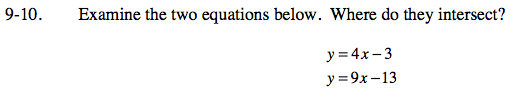### Home > CCA > Chapter 9 > Lesson 9.1.1 > Problem9-10

9-10.

Examine the two equations below. Where do they intersect? Homework Help ✎

 y = 4x − 3 y = 9x − 13There are many ways to solve this problem. You could use a table, a graph, the Equal Values method, the Substitution method, or the Elimination method.